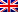#[Sharif University CTF 2016] [Forensic 50 – Kick Tort Teen] Write Up

Anagram, anyone?

## Resolution

We had a hint : Anagram, and a .xls file, we download it, we see w columns containing numbers with 2 to 3 digits. There are around 15k rows. We export the .xls to a csv for easier parsing and then do some analysis. We find out that there are only 256 unique numbers, interesting huh ? 🙂 That sounds like they represent byte numbers. If we look at the top of the document, most of the first numbers are all 78, the 4 first numbers are different than 78. So it could be a binary format. We analyse a bit more the numbers, they start at 78 and finish at 78 + 256 * 3. We send make a little C# script to transform the file in a binary.

Here it is:

```using System;
using System.IO;

class Solution
{
static void Main(String[] args)
{
byte[] arr = new byte;
int i = 0;
foreach (string s in data)
{
String[] tmp = s.Split(';');
// each number
foreach (string z in tmp)
{
int n = Convert.ToInt32(z);
n = n - 78;
if (n > 0)
n /= 3;
arr[i] = Convert.ToByte(n);
i++;
}
}
File.WriteAllBytes(@"G:\Users\laxa\Desktop\bla.bin", arr);
Console.WriteLine("Done!");
```\$ file bla.bin